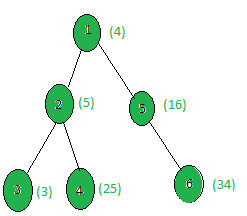# Count the nodes whose sum with X is a Fibonacci number

Given a tree, and the weights of all the nodes and an integer X, the task is to count all the nodes i such that (weight[i] + X) is a Fibonacci Number.

First few fibonacci numbers are:

0, 1, 1, 2, 3, 5, 8, 13, 21, 34, 55, 89, 141, …

Examples:

Input:X = 5
Output: 2
Only the nodes 3 and 5 give a fibonacci number when 5 is added to them.
i.e. (3 + 5) = 8 and (16 + 5) = 21 are both Fibonacci numbers.

## Recommended: Please try your approach on {IDE} first, before moving on to the solution.

Approach: Perform dfs on the tree and count all the nodes sum of whose weight with x is a fibonacci number.

Below is the implementation of the above approach:

 `// C++ implementation of the approach ` `#include ` `using` `namespace` `std; ` ` `  `int` `ans = 0, x; ` ` `  `vector<``int``> graph; ` `vector<``int``> weight(100); ` ` `  `// Function that returns true if ` `// x is a perfect square ` `bool` `isPerfectSquare(``long` `double` `x) ` `{ ` `    ``// Find floating point value of ` `    ``// square root of x ` `    ``long` `double` `sr = ``sqrt``(x); ` ` `  `    ``// If square root is an integer ` `    ``return` `((sr - ``floor``(sr)) == 0); ` `} ` ` `  `// Function that returns true ` `// if n is a fibonacci number ` `bool` `isFibonacci(``int` `n) ` `{ ` `    ``return` `isPerfectSquare(5 * n * n + 4) ` `           ``|| isPerfectSquare(5 * n * n - 4); ` `} ` ` `  `// Function to perform dfs ` `void` `dfs(``int` `node, ``int` `parent) ` `{ ` `    ``// If weight of the current node ` `    ``// gives a fibonacci number ` `    ``// when x is added to it ` `    ``if` `(isFibonacci(weight[node] + x)) ` `        ``ans += 1; ` ` `  `    ``for` `(``int` `to : graph[node]) { ` `        ``if` `(to == parent) ` `            ``continue``; ` `        ``dfs(to, node); ` `    ``} ` `} ` ` `  `// Driver code ` `int` `main() ` `{ ` `    ``x = 5; ` ` `  `    ``// Weights of the node ` `    ``weight = 4; ` `    ``weight = 5; ` `    ``weight = 3; ` `    ``weight = 25; ` `    ``weight = 16; ` `    ``weight = 34; ` ` `  `    ``// Edges of the tree ` `    ``graph.push_back(2); ` `    ``graph.push_back(3); ` `    ``graph.push_back(4); ` `    ``graph.push_back(5); ` `    ``graph.push_back(6); ` ` `  `    ``dfs(1, 1); ` ` `  `    ``cout << ans; ` ` `  `    ``return` `0; ` `} `

Output:

```2
```

Attention reader! Don’t stop learning now. Get hold of all the important DSA concepts with the DSA Self Paced Course at a student-friendly price and become industry ready.

My Personal Notes arrow_drop_upCheck out this Author's contributed articles.

If you like GeeksforGeeks and would like to contribute, you can also write an article using contribute.geeksforgeeks.org or mail your article to contribute@geeksforgeeks.org. See your article appearing on the GeeksforGeeks main page and help other Geeks.

Please Improve this article if you find anything incorrect by clicking on the "Improve Article" button below.

Article Tags :
Practice Tags :

Be the First to upvote.

Please write to us at contribute@geeksforgeeks.org to report any issue with the above content.